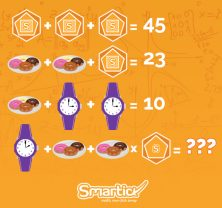Smartick is a fun way to learn math!Apr27

# Do You Dare to Try and Solve this Math Riddle?When we received this math puzzle, we shared it in the Whatsapp group we have for the whole Smartick team. When the answers started to come back, they were all different!

Given that we have so many whizzes working here at Smartick (developers, mathematicians, physicists, engineers), it was surprising that it took so long to find the right answer. Do you dare to try it yourself? It seems easy, just another one of the many puzzles that are shared daily on social networks, but this one is trickier than it seems so watch out! Pay close attention to the details, and post your answer in the comments section if you’re brave enough.

****Stop reading here if you want to try it yourself!****

Here are a few clues you can use to make sure you’re on the right track:

• Look closely at the shape that frames the S of our logo. It’s a square within a pentagon within a hexagon. How many sides do these 3 geometric figures have in total? Is the shape the same on all the lines?
• Are there the same number of doughnuts on all the plates?
• What time is shown on the clock?
• And after so many tricky details, one more. There are two different operations on the last line: addition and multiplication. Do you remember how to do combined operations? Here’s a post from our blog where you can see a quick review of combined operations.

I’m sure you’ll get the right answer using all the clues!

Here’s the solution, so you can check whether you’ve done it right.

LINE 1: 15 + 15 + 15 = 45

Or, 45 ÷ 3 = 15, which is the same

Note that each 15 is made up of a square (4 sides), a pentagon (5 sides), and a hexagon (6 sides): 4 + 5 + 6 = 15

LINE 2: 4 + 4 + 15 = 23

Note that there are doughnuts on each plate.

LINE 3: 4 + 3 + 3 = 10

Each clock reads 3 o’clock, or 3 hours.

LINE 4: 2 + 3 + 3 x 11 = 38

This is where you will have gone wrong if you haven’t paid attention. The clock shows 2 instead of 3. There are 3 doughnuts on each plate instead of 4. The shape around the S of our logo is different from line 1, this one only contains a pentagon (5 sides) and a hexagon (6 sides). The sum of the sides of these two figures is 11 since 5 + 6 = 11. And the multiplication is done before the addition!

3 x 11 = 33

2 + 3 + 33 = 38

If you’ve avoided all the traps, I’m sure you’ll have got the same result as me for this math puzzle.

See how fun math can be?!

Fun is our brain’s favorite way of learning
Diane Ackerman
Smartick is a fun way to learn math
• 15 fun minutes a day
• Millions of students since 2009• anomuosSep 14 2021, 9:50 AM

the answer is 88 or 165 but i think its 88